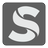Area of a Convex Polygon
Simple+
English ES RU

There is a convex polygon on a coordinate plane. This polygon is presented as a list of vertices coordinates. Each vertex is connected sequentially with the last connecting back to the first. A polygon has N vertices. You should write a program that will calculate the area of a polygon. The result should be given with one digits precision as ±0.1.Input: Coordinates as a list of lists. Each list contains two integers.

Output: The area of the polygon as a float.

Example:

```checkio([[1, 1], [9, 9], [9, 1]]) == 32
checkio([[4, 10], [7, 1], [1, 4]]) == 22.5
checkio([[1, 2], [3, 8], [9, 8], [7, 1]]) == 40
checkio([[3, 3], [2, 7], [5, 9], [8, 7], [7, 3]]) == 26
checkio([[7, 2], [3, 2], [1, 5],
[3, 9], [7, 9], [9, 6]]) == 42
checkio([[4, 1], [3, 4], [3, 7], [4, 8],
[7, 9], [9, 6], [7, 1]]) == 35.5```

How it is used: This concept is very useful in topography and architecture. You can improve the algorithm and calculate the area of any zone on the map taking into account elevation and other points of interest. You could also use this idea to construct something like a building, or a boat.

Precondition: 3 ≤ N ≤ 8
∀ x, y ∈ coordinates : 0 ≤ x ≤ 10; 0 ≤ y ≤ 10;